Courses

# Tables - Practice Test (With Solutions) CAT, Common Admission Test

## 13 Questions MCQ Test | Tables - Practice Test (With Solutions) CAT, Common Admission Test

Description
This mock test of Tables - Practice Test (With Solutions) CAT, Common Admission Test for LR helps you for every LR entrance exam. This contains 13 Multiple Choice Questions for LR Tables - Practice Test (With Solutions) CAT, Common Admission Test (mcq) to study with solutions a complete question bank. The solved questions answers in this Tables - Practice Test (With Solutions) CAT, Common Admission Test quiz give you a good mix of easy questions and tough questions. LR students definitely take this Tables - Practice Test (With Solutions) CAT, Common Admission Test exercise for a better result in the exam. You can find other Tables - Practice Test (With Solutions) CAT, Common Admission Test extra questions, long questions & short questions for LR on EduRev as well by searching above.
QUESTION: 1

### Refer to th e following table and solve the questions based on it, The following table gives the exports of top six industries (in value terms) from India in 2007: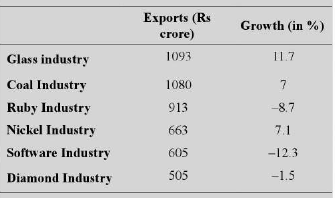The overall exports from India increased by 20% to Rs 19,500 crore m 2007 What is the share of the Glass industry in the total exports for 2007?

Solution:

The share of Glass in the total exports for 2007 = 1093/19500 x 100 = 5.6%.

QUESTION: 2

### Refer to th e following table and solve the questions based on it, The following table gives the exports of top six industries (in value terms) from India in 2007:The overall exports from India increased by 20% to Rs 19,500 crore m 2007 Q. Which of the following has the maximum share among the given industries in 2006?

Solution:

Coal has the maximum exports in 2006. Hence, its market share is the maximum.

QUESTION: 3

### Refer to the following table and solve the questions based on it. The following table gives the number of vehicles (in v000s) of different models and colours sold in two cities— Patna and Lucknow-in a given year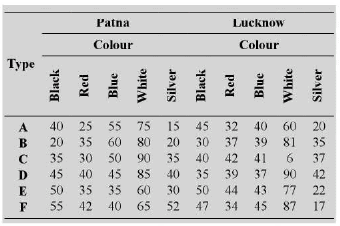For which of the following models, difference between white—coloured vehicles sold in the two cities is maximum?

Solution:

The difference between the white-coloured cars sold is the maximum in the C type model.

QUESTION: 4

R efer to th e following table and solve the questions based on it.
The following table gives the number of vehicles (in v000s) of different models and colours sold in two cities— Patna and Lucknow-in a given yearWhat is the difference between the number of blue- coloured vehicles of model C sold in Patna and the number of red coloured vehicles of model F sold in Lucknow?

Solution:

Required difference = (50 - 34) x 1000 = 16,000

QUESTION: 5

R efer to th e following table and solve the questions based on it.
The following table gives the number of vehicles (in v000s) of different models and colours sold in two cities— Patna and Lucknow-in a given yearIn Patna the number of vehicles sold was the maximum for which of the colour-model combinations?

Solution:

White-c = 90,000

QUESTION: 6

Refer to the following table and solve the questions based on it The table given below gives the marks obtained by six students in six subjects: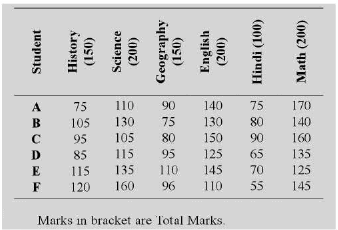The marks obtained by student A in Math are how many times the percentage marks obtained by student F in Science?

Solution:

he percentage of marks obtained by F in Science = 160/200 x 100 = 80% Required Value = 170/80 = 2.125

QUESTION: 7

Refer to the following table and solve the questions based on it The table given below gives the marks obtained by six students in six subjects:Approximately, what is the average percentage marks obtained by the six students in English?

Solution: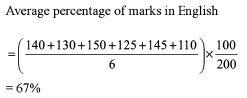QUESTION: 8

Refer to the following table and solve the questions based on i t The following table gives the average marks obtained by 20 boys and 20 girls in five subjects from five different schools Pn Q. R. S and T: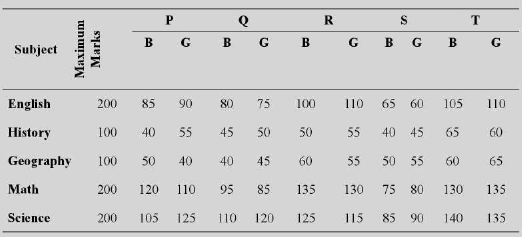In the above table, B = Bovs and G = Girls

What were the total marks obtained by the boys in History from school Q?

Solution:

Average marks obtained by 20 boys in History from school Q = 45 Therefore, Total marks = 20 x 45 = 900

QUESTION: 9

Refer to the following table and solve the questions based on it The following table gives the average marks obtained by 20 boys and 20 girls in five subjects from five different schools Pn Q. R. S and T:In the above table, B = Bovs and G = Girls

What is the average mark obtained by all the students of school P taking all the subjects into account?

Solution:

The table gives the data pertaining to only 40 students of the school and it is not given if this is the total number of students in the school. Therefore it cannot be determined

QUESTION: 10

Refer to the following table and solve the questions based on it.
The following table shows the domestic sales of cars of five manufacturers from 1995 to 2000. (All the figures are in thousands)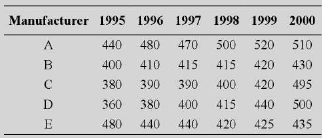Considering the period given, the domestic sales of which manufacturer are the highest?

Solution:

It is a simple calculation. Alternatively, it can be observed that A has always been more than the others except but for one year.

QUESTION: 11

Refer to the following table and solve the questions based on it.
The following table shows the domestic sales of cars of five manufacturers from 1995 to 2000. (All the figures are in thousands)Q.

During 2000, the sales of which manufacturer has shown the maximum percentage increase over the previous year?

Solution:

It can be observed that the difference is the highest in case of manufacturer C. We can further observe that the largest percentage growth would be for C, as the base is the smallest.

QUESTION: 12

Refer to the following table and solve the questions based on it.
The following table shows the domestic sales of cars of five manufacturers from 1995 to 2000. (All the figures are in thousands)Which of the following manufacturers, has a consistent increase across the years given?

Solution:

By visual inspection we can say that option (d) is the correct answer.

QUESTION: 13

Refer to the following table and solve the questions based on it.
The following table shows the domestic sales of cars of five manufacturers from 1995 to 2000. (All the figures are in thousands)Q.

How many times have the number of cars sold been more than 450 for any manufacturer in any year more than once? (A manufacturer can achieve this feat)

Solution:

By visual inspection we can say that option (d) is the correct answer.